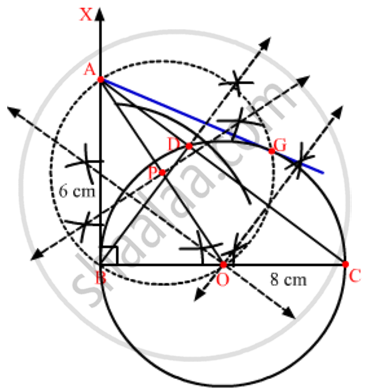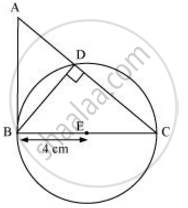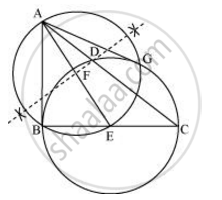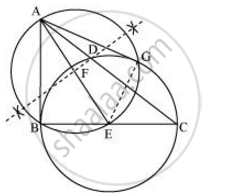# Draw a right triangle ABC in which AB = 6 cm, BC = 8 cm and ∠B = 90°. Draw BD perpendicular from B on AC and draw a circle passing through the points B, C and D. Construct tangents - Mathematics

Draw a right triangle ABC in which AB = 6 cm, BC = 8 cm and ∠B = 90°. Draw BD perpendicular from B on AC and draw a circle passing through the points B, C and D. Construct tangents from A to this circle.

Let ABC be a right triangle in which AB = 6 cm, BC = 8 cm and ∠B = 90°. BD is the perpendicular from B on AC. The circle through B, C, and D is drawn. Construct the tangents from A to this circle. Give the justification of the construction.

#### Solution 1

Follow the given steps to construct the figure.
Step 1
Draw a line BC of 8 cm length.
Step 2
Draw BX perpendicular to BC.
Step 3
Mark an arc at the distance of 6 cm on BX. Mark it as A.
Step 4
Join A and C. Thus, ∆ABC is the required triangle.
Step 5
With B as the centre, draw an arc on AC.
Step 6
Draw the bisector of this arc and join it with B. Thus, BD is perpendicular to AC.
Step 7
Now, draw the perpendicular bisector of BD and CD. Take the point of intersection as O.
Step 8
With O as the centre and OB as the radius, draw a circle passing through points B, C and D.
Step 9
Join A and O and bisect it. Let P be the midpoint of AO.
Step 10
Taking P as the centre and PO as its radius, draw a circle which will intersect the circle at point B and G. Join A and G.
Here, AB and AG are the required tangents to the circle from A.#### Solution 2

Consider the following situation. If a circle is drawn through B, D, and C, BC will be its diameter as ∠BDC is of measure 90°. The centre E of this circle will be the mid-point of BC.The required tangents can be constructed on the given circle as follows.

Step 1

Join AE and bisect it. Let F be the mid-point of AE.

Step 2

Taking F as centre and FE as its radius, draw a circle which will intersect the circle at point B and G. Join AG.

AB and AG are the required tangents.Justification

The construction can be justified by proving that AG and AB are the tangents to the circle. For this, join EG.∠AGE is an angle in the semi-circle. We know that an angle in a semi-circle is a right angle.

∴ ∠AGE = 90°

⇒ EG ⊥ AG

Since EG is the radius of the circle, AG has to be a tangent of the circle.

⇒ AB ⊥ BE

Since BE is the radius of the circle, AB has to be a tangent of the circle.

Concept: Construction of Tangents to a Circle
Is there an error in this question or solution?
Chapter 11: Constructions - Exercise 11.2 [Page 222]

#### APPEARS IN

NCERT Class 10 Maths
Chapter 11 Constructions
Exercise 11.2 | Q 6 | Page 222
RD Sharma Class 10 Maths
Chapter 9 Constructions
Exercise 9.3 | Q 6 | Page 18
Share